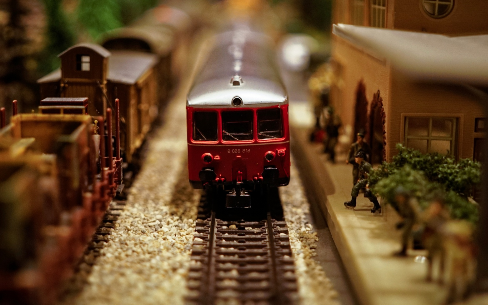# Definition of ModelA model is a three-dimensional representation of a person or thing.

Models are often smaller or simpler than the original object.

The picture shows some model trains.

In mathematics, we also create models, but of a different kind. They use equations and computer programs to try to simulate the behaviour of processes or experiments in the real world. Some things need to be simplified so that the model will return results in real time, but mathematical models can be very useful for testing hypotheses and making predictions about the effects of small changes in temperature, prices, wind speed and so on.

### Description

The aim of this dictionary is to provide definitions to common mathematical terms. Students learn a new math skill every week at school, sometimes just before they start a new skill, if they want to look at what a specific term means, this is where this dictionary will become handy and a go-to guide for a student.

### Audience

Year 1 to Year 12 students

### Learning Objectives

Learn common math terms starting with letter M

Author: Subject Coach
You must be logged in as Student to ask a Question.